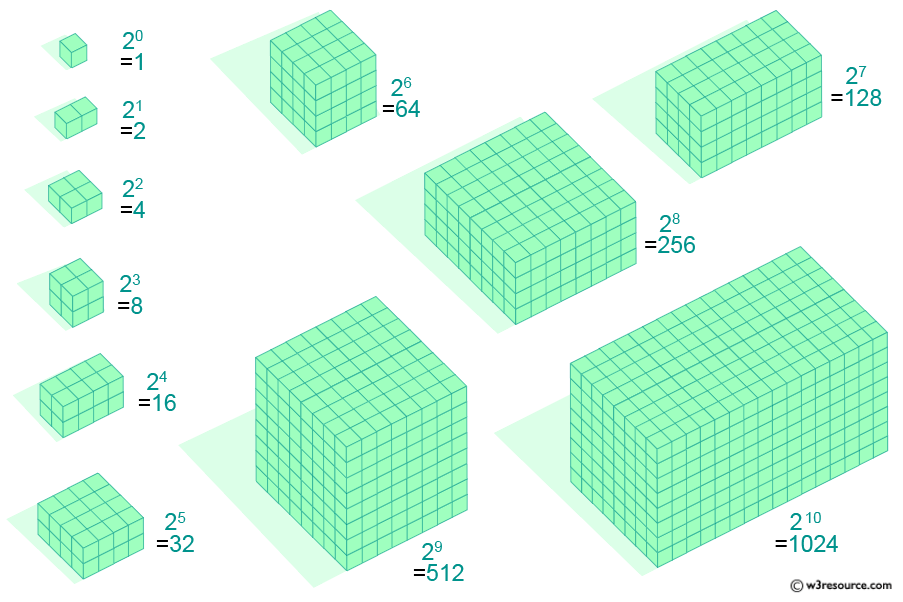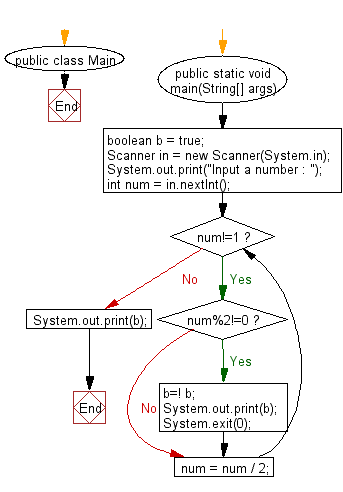# Java: Check whether an given integer is power of 2 or not using O(1) time

## Java Basic: Exercise-205 with Solution

Write a Java program to check whether an integer is a power of 2 or not using O(1) time.

Note: O(1) means that it takes a constant time, like 12 nanoseconds, or two minutes no matter the amount of data in the set.
O(n) means it takes an amount of time linear with the size of the set, so a set twice the size will take twice the time. You probably don't want to put a million objects into one of these.

Visualization of powers of two from 1 to 1024:Sample Solution:

Java Code:

``````import java.util.*;
public class Main
{
public static void main(String[] args)
{
boolean b = true;
Scanner in = new Scanner(System.in);
System.out.print("Input a number : ");
int num = in.nextInt();
{
while(num!=1)
{
if(num%2!=0)
{
b=! b;
System.out.print(b);
System.exit(0);
}
num = num / 2;
}
System.out.print(b);
}
}

}
```
```

Sample Output:

```Input a number :  25
false
```

Flowchart:Java Code Editor: